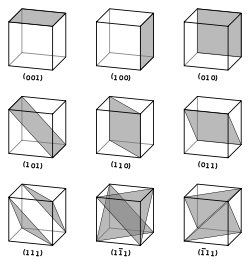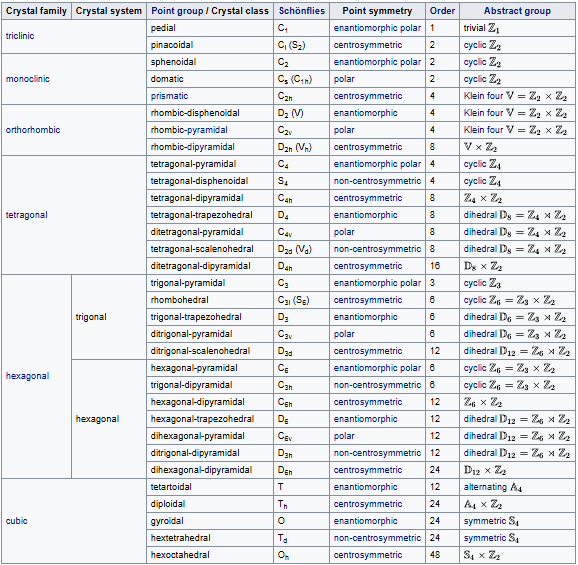# Classification of crystalline symmetries

Author:
Event:

Visualization(s): 396

Created: 2018-10-07 19:27:44.

External Link: Classification of crystalline symmetries

Resume:
Videos 0 Images 3 Audios 0 Files 0 Links 0
Tags:

No tags ...

Crystal structure is a description of the ordered arrangement of atoms, ions or molecules in a crystalline material. Ordered structures occur from the intrinsic nature of the constituent particles to form symmetric patterns that repeat along the principal directions of three-dimensional space in matter. The smallest group of particles in the material that constitutes the repeating pattern is the unit cell of the structure. The unit cell completely defines the symmetry and structure of the entire crystal lattice, which is built up by repetitive translation of the unit cell along its principal axes. The repeating patterns are said to be located at the points of the Bravais lattice.

The lengths of the principal axes, or edges, of the unit cell and the angles between them are the lattice constants, also called lattice parameters. The symmetry properties of the crystal are described by the concept of space groups. All possible symmetric arrangements of particles in three-dimensional space may be described by the 230 space groups. The crystal structure and symmetry play a critical role in determining many physical properties, such as cleavage, electronic band structure, and optical transparency.

Crystal structure is described in terms of the geometry of arrangement of particles in the unit cell. The unit cell is defined as the smallest repeating unit having the full symmetry of the crystal structure. The geometry of the unit cell is defined as a parallelepiped, providing six lattice parameters taken as the lengths of the cell edges (a, b, c) and the angles between them (α, β, γ). The positions of particles inside the unit cell are described by the fractional coordinates (xi, yi, zi) along the cell edges, measured from a reference point. It is only necessary to report the coordinates of a smallest asymmetric subset of particles. This group of particles may be chosen so that it occupies the smallest physical space, which means that not all particles need to be physically located inside the boundaries given by the lattice parameters. All other particles of the unit cell are generated by the symmetry operations that characterize the symmetry of the unit cell. The collection of symmetry operations of the unit cell is expressed formally as the space group of the crystal structure.

Vectors and planes in a crystal lattice are described by the three-value Miller index notation. This syntax uses the indices ℓ, m, and n as directional orthogonal parameters, which are separated by 90°.

By definition, the syntax (ℓmn) denotes a plane that intercepts the three points a1/ℓ, a2/m, and a3/n, or some multiple thereof. That is, the Miller indices are proportional to the inverses of the intercepts of the plane with the unit cell (in the basis of the lattice vectors). If one or more of the indices is zero, it means that the planes do not intersect that axis (i.e., the intercept is "at infinity"). A plane containing a coordinate axis is translated so that it no longer contains that axis before its Miller indices are determined. The Miller indices for a plane are integers with no common factors. Negative indices are indicated with horizontal bars, as in (123). In an orthogonal coordinate system for a cubic cell, the Miller indices of a plane are the Cartesian components of a vector normal to the plane.

Considering only (ℓmn) planes intersecting one or more lattice points (the lattice planes), the distance d between adjacent lattice planes is related to the (shortest) reciprocal lattice vector orthogonal to the planes by the formula

### D = 2π/|glmn|Planes with different Miller indices in cubic crystals.

The crystallographic directions are geometric lines linking nodes (atoms, ions or molecules) of a crystal. Likewise, the crystallographic planes are geometric planes linking nodes. Some directions and planes have a higher density of nodes. These high density planes have an influence on the behavior of the crystal as follows:

• Optical properties: Refractive index is directly related to density (or periodic density fluctuations).
• Adsorption and reactivity: Physical adsorption and chemical reactions occur at or near surface atoms or molecules. These phenomena are thus sensitive to the density of nodes.
• Surface tension: The condensation of a material means that the atoms, ions or molecules are more stable if they are surrounded by other similar species. The surface tension of an interface thus varies according to the density on the surface.
• Microstructural defects: Pores and crystallites tend to have straight grain boundaries following higher density planes.
• Cleavage: This typically occurs preferentially parallel to higher density planes.
• Plastic deformation: Dislocation glide occurs preferentially parallel to higher density planes. The perturbation carried by the dislocation (Burgers vector) is along a dense direction. The shift of one node in a more dense direction requires a lesser distortion of the crystal lattice.

Some directions and planes are defined by symmetry of the crystal system. In monoclinic, rhombohedral, tetragonal, and trigonal/hexagonal systems there is one unique axis (sometimes called the principal axis) which has higher rotational symmetry than the other two axes. The basal plane is the plane perpendicular to the principal axis in these crystal systems. For triclinic, orthorhombic, and cubic crystal systems the axis designation is arbitrary and there is no principal axis.

The defining property of a crystal is its inherent symmetry, by which we mean that under certain 'operations' the crystal remains unchanged. All crystals have translational symmetry in three directions, but some have other symmetry elements as well. For example, rotating the crystal 180° about a certain axis may result in an atomic configuration that is identical to the original configuration. The crystal is then said to have a twofold rotational symmetry about this axis. In addition to rotational symmetries like this, a crystal may have symmetries in the form of mirror planes and translational symmetries, and also the so-called "compound symmetries," which are a combination of translation and rotation/mirror symmetries. A full classification of a crystal is achieved when all of these inherent symmetries of the crystal are identified.

#### Lattice systems

These lattice systems are a grouping of crystal structures according to the axial system used to describe their lattice. Each lattice system consists of a set of three axes in a particular geometric arrangement. There are seven lattice systems. They are similar to but not quite the same as the seven crystal systems.

The simplest and most symmetric, the cubic (or isometric) system, has the symmetry of a cube, that is, it exhibits four threefold rotational axes oriented at 109.5° (the tetrahedral angle) with respect to each other. These threefold axes lie along the body diagonals of the cube. The other six lattice systems, are hexagonal, tetragonal, rhombohedral (often confused with the trigonal crystal system), orthorhombic, monoclinic and triclinic.

#### Bravais lattices

Bravais lattices, also referred to as space lattices, describe the geometric arrangement of the lattice points, and therefore the translational symmetry of the crystal. The three dimensions of space afford 14 distinct Bravais lattices describing the translational symmetry. All crystalline materials recognized today, not including quasicrystals, fit in one of these arrangements. The fourteen three-dimensional lattices, classified by lattice system, are shown above.

The crystal structure consists of the same group of atoms, the basis, positioned around each and every lattice point. This group of atoms therefore repeats indefinitely in three dimensions according to the arrangement of one of the Bravais lattices. The characteristic rotation and mirror symmetries of the unit cell is described by its crystallographic point group.

In geometry and crystallography, a Bravais lattice is a category of symmetry groups for translational symmetry in three directions, or correspondingly, a category of translation lattices.

Such symmetry groups consist of translations by vectors of the form

#### R = n1a1 + n2a2 + n3a3

where n1, n2, and n3 are integers and a1, a2, and a3 are three non-coplanar vectors, called primitive vectors.

These lattices are classified by space group of the translation lattice itself; there are 14 Bravais lattices in three dimensions; each can apply in one lattice system only. They represent the maximum symmetry a structure with the translational symmetry concerned can have. All crystalline materials must, by definition fit in one of these arrangements (not including quasicrystals).

For convenience a Bravais lattice is depicted by a unit cell which is a factor 1, 2, 3 or 4 larger than the primitive cell. Depending on the symmetry of a crystal or other pattern, the fundamental domain is again smaller, up to a factor 48. The Bravais lattices were studied by Moritz Ludwig Frankenheim in 1842, who found that there were 15 Bravais lattices. This was corrected to 14 by A. Bravais in 1848.Crystalline symmetries.

#### Crystal systems

A crystal system is a set of point groups in which the point groups themselves and their corresponding space groups are assigned to a lattice system. Of the 32 point groups that exist in three dimensions, most are assigned to only one lattice system, in which case the crystal system and lattice system both have the same name. However, five point groups are assigned to two lattice systems, rhombohedral and hexagonal, because both lattice systems exhibit threefold rotational symmetry. These point groups are assigned to the trigonal crystal system.Crystal systems.

In total there are seven crystal systems: triclinic, monoclinic, orthorhombic, tetragonal, trigonal, hexagonal, and cubic.

REFERENCES

Wikipedia. Available in: https://en.wikipedia.org/wiki/Crystal_structure. Access in: 07/10/2018.

Wikipedia. Available in: https://en.wikipedia.org/wiki/Bravais_lattice. Access in: 07/10/2018.

Wikipedia. Available in: https://en.wikipedia.org/wiki/Crystal_system. Access in: 07/10/2018.

##### Physics

Default timespace